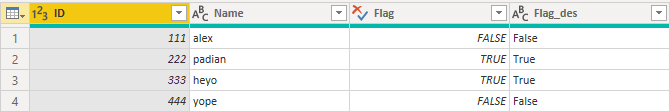cancel
Showing results for
Did you mean:Anonymous
Not applicable

## How to use R script in power query ? for populating Column using if condition in R SCRIPT

Hi

I have a table A(exist in power bi data model)

 Table A ID Name Flag 111 alex F 222 padian T 333 heyo T 444 yope F

I want to create a new column 'Flad des' using 'Flag column' by if condition in R SCRIPT, find the below exepected output for your reference.
O/P:

 Table A ID Name Flag Flag des 111 alex F FALSE 222 padian T TRUE 333 heyo T TRUE 444 yope F FALSE

If you could help me it would be helpfull, also please share me the screehot if possible .

1 ACCEPTED SOLUTIONCommunity Support

Hi @Anonymous ,

``````
dataset\$Flag_des <- ifelse(dataset\$Flag,"True","False")
output <- dataset``````

``````let
Source = Table.FromRows(Json.Document(Binary.Decompress(Binary.FromText("i45WMjQ0VNJRSsxJrQBSbkqxOtFKRkZGQHZBYkpmYh6QEQIWNDY2BrIzUivz4UImJiZAdmV+QSpEaywA", BinaryEncoding.Base64), Compression.Deflate)), let _t = ((type nullable text) meta [Serialized.Text = true]) in type table [ID = _t, Name = _t, Flag = _t]),
#"Changed Type" = Table.TransformColumnTypes(Source,{{"ID", Int64.Type}, {"Name", type text}, {"Flag", type text}}),
#"Run R script" = R.Execute("# 'dataset' holds the input data for this script#(lf)  #(lf)dataset\$Flag_des <- ifelse(dataset\$Flag ,""True"",""False"")#(lf)output <- dataset",[dataset=#"Changed Type"]),
#"""output""" = #"Run R script"{[Name="output"]}[Value]
in
#"""output"""``````Best Regards,
Xue Ding
If this post helps, then please consider Accept it as the solution to help the other members find it more quickly.
2 REPLIES 2Community Support

Hi @Anonymous ,

``````
dataset\$Flag_des <- ifelse(dataset\$Flag,"True","False")
output <- dataset``````

``````let
Source = Table.FromRows(Json.Document(Binary.Decompress(Binary.FromText("i45WMjQ0VNJRSsxJrQBSbkqxOtFKRkZGQHZBYkpmYh6QEQIWNDY2BrIzUivz4UImJiZAdmV+QSpEaywA", BinaryEncoding.Base64), Compression.Deflate)), let _t = ((type nullable text) meta [Serialized.Text = true]) in type table [ID = _t, Name = _t, Flag = _t]),
#"Changed Type" = Table.TransformColumnTypes(Source,{{"ID", Int64.Type}, {"Name", type text}, {"Flag", type text}}),
#"Run R script" = R.Execute("# 'dataset' holds the input data for this script#(lf)  #(lf)dataset\$Flag_des <- ifelse(dataset\$Flag ,""True"",""False"")#(lf)output <- dataset",[dataset=#"Changed Type"]),
#"""output""" = #"Run R script"{[Name="output"]}[Value]
in
#"""output"""``````Best Regards,
Xue Ding
If this post helps, then please consider Accept it as the solution to help the other members find it more quickly.Super User

@Anonymous -Become an expert!: Enterprise DNA
External Tools: MSHGQM
Latest book!:
Mastering Power BI 2nd EditionDAX is easy, CALCULATE makes DAX hard...